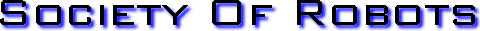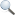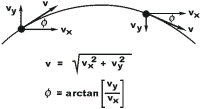Search Here MISC  Parts List  Robot Forum  Chat  Member Pages  Axon MCU  Robot Books  Shop  Contact HARDWARE  Actuators  Batteries  Electronics  Materials  Microcontrollers  Sensors SCIENCE  Robot Journals  Robot Theory  Conferences PROGRAMMING - TRIGONOMETRY LOOKUP TABLETrigonometric Lookup Tables were used quite often way back when computers were only in labs and not everyday in people's homes. Back then computers were slow, so slow that it would take some significant amount of time to solve say, cos(40). And processors were unable to do it through hardware too. So if you needed to solve trigonometry functions real time, you would need a different solution. Yes you guessed it, a lookup table. Why would you need this? Because although computers can do billions of trig functions in seconds, that little tiny PIC you have on your omni-wheel robot cannot. So here is how to do it. Copy/paste my PIC C code below and change the syntax to whatever language you are using. Too easy, huh? =P To call the function just say: output=anglookuptable(the_angle_in_degrees,0);// 0 for cosine, 1 for sine The Trigonometric Lookup Table Code: signed int angtable={100,99,94,87,77,64,50,34,17,0}; //multiplied by 100 so no floating point math //trig lookup table, type 1 for cos, 0 for sin, degrees is from 0->360 signed int anglookuptable(long int degrees, int type); //trig lookup table, type 0 for cos, 1 for sin, degrees is from 0->360 signed int anglookuptable(long int degrees, int type) { //{100,99,94,87,77,64,50,34,17,0} signed int c=1; signed int s=1; int i=(degrees/10); //includes 0 to 90 degrees if (i > 9 && i < = 18) //between 91 and 180 { i=18-i; c=-1; } if (i > 18 && i< = 27) //between 181 and 270 { i=i-18; c=-1; s=-1; } if (i > 27 && i < =36) //between 271 and 360 { i=36-i; s=-1; } if (type==1) //cosine { //printf("%d\r\n",c*angtable[i]); return c*angtable[i]; } else //sine { //printf("%d\r\n",s*angtable[9-i]); return s*angtable[9-i]; } } Get Your Ad Here Has this site helped you with your robot? Give us credit - link back, and help others in the forums!Society of Robots copyright 2005-2014 forum SMF post simple machines